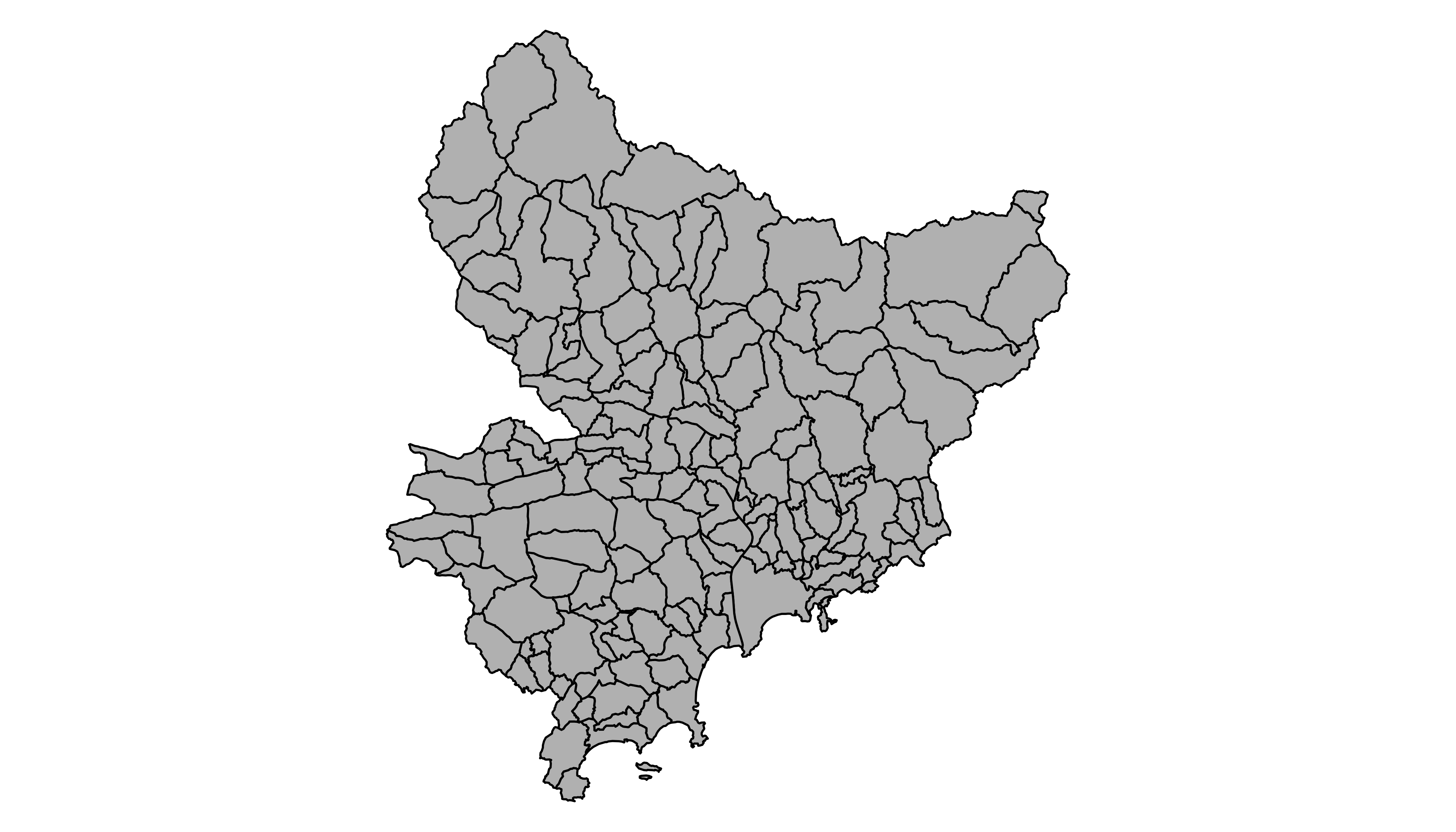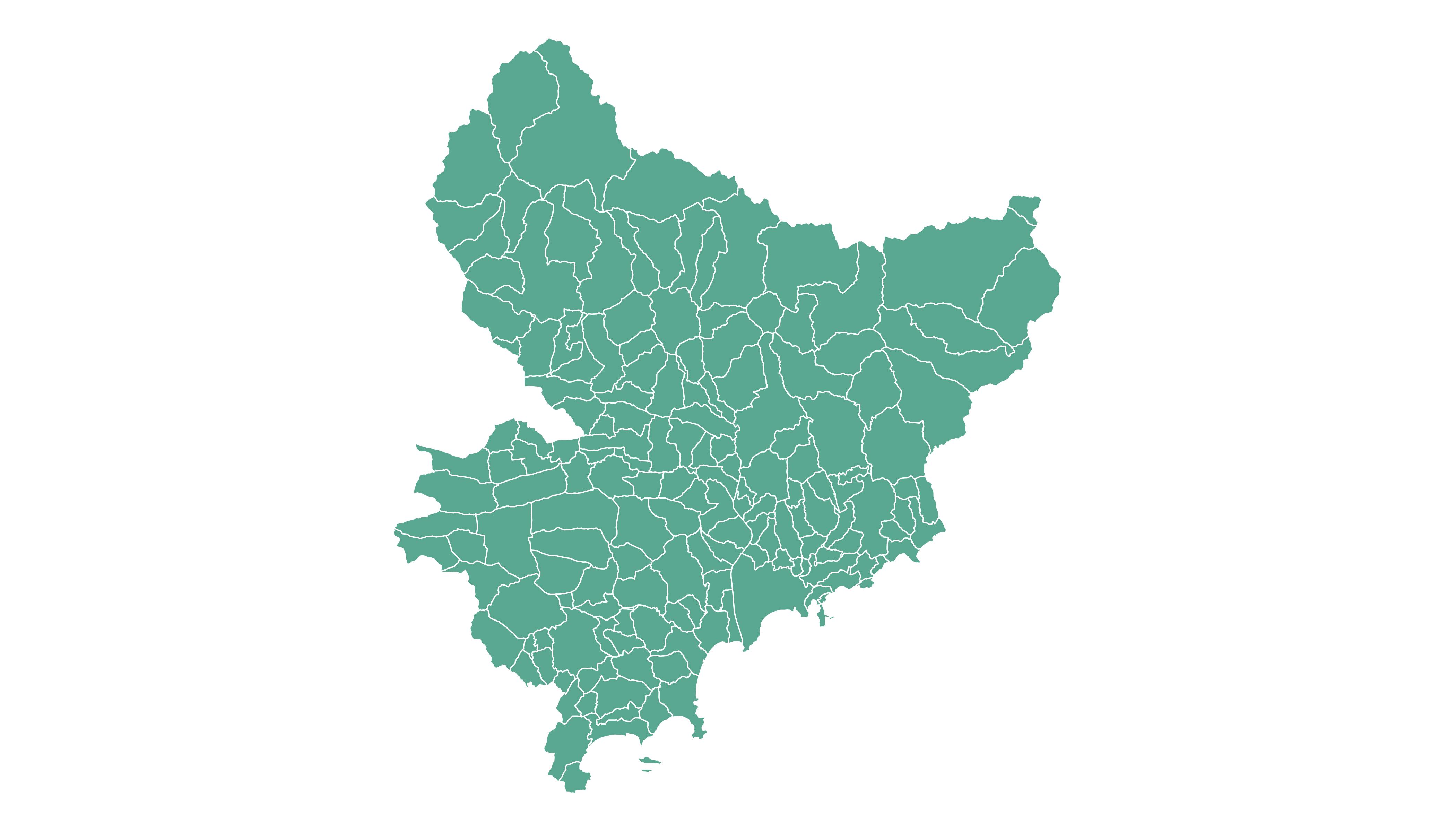# Open and Plot Geojson files in R

Geojson files are a common way to store geospatial data. This post explains how to read it with `R` and the `geojsonio` package, and how to plot it in base R or with `ggplot2`.

If you did not find the geospatial data you need in existing R packages (see the map section), you need to find this information elsewhere on the web.

It will often be stored as a `.geomJSON` format. This post explains how to read it.

Note: if you found a `shapefile`, read this post instead.

# Find and download a `.geoJSON` file

You need to dig the internet to find the geoJSON file you are interested in. For instance, this URL provides a file containing french region boundaries.

You can load it in R with:

``````# Let's read the jeoJson file that is stored on the web with the geojsonio library:
library(geojsonio)
spdf <- geojson_read("https://raw.githubusercontent.com/gregoiredavid/france-geojson/master/communes.geojson",  what = "sp")``````

That’s it! You now have a geospatial object called `spdf`. I strongly advise to read this post to learn how to manipulate it.

Just in case, here is how to plot it in base R and with `ggplot2`.

# Plot it with `base R`

The basic `plot()` function knows how to plot a geospatial object. Thus you just need to pass it `spdf` and add a couple of options to customize the output.``````# Select only the region #6
spdf@data\$mystate = substr( spdf@data\$code, 1, 2)
spdf_region_6 = spdf[ spdf@data\$mystate == "06" , ]

# plot the selected are with sp
library(sp)
par(mar=c(0,0,0,0))
plot(spdf_region_6, col="grey")``````

# Plot it with `ggplot2`

It is totally possible (and advised imo) to build the map with ggplot2. However, ggplot2 takes as input data frames, not geospatial data.

The geospatial object thus needs to be transformed using the `tidy()` function of the `broom` package.

Once the data frame is created, it is plotted using the `geom_polygon()` function as described below.``````# 'fortify' the data to get a dataframe format required by ggplot2
library(broom)
spdf_fortified <- tidy(spdf_region_6)

# Plot it
library(ggplot2)
ggplot() +
geom_polygon(data = spdf_fortified, aes( x = long, y = lat, group = group), fill="#69b3a2", color="white") +
theme_void() +
coord_map()``````

Related chart types

## Contact

This document is a work by Yan Holtz. Any feedback is highly encouraged. You can fill an issue on Github, drop me a message on Twitter, or send an email pasting yan.holtz.data with gmail.com.